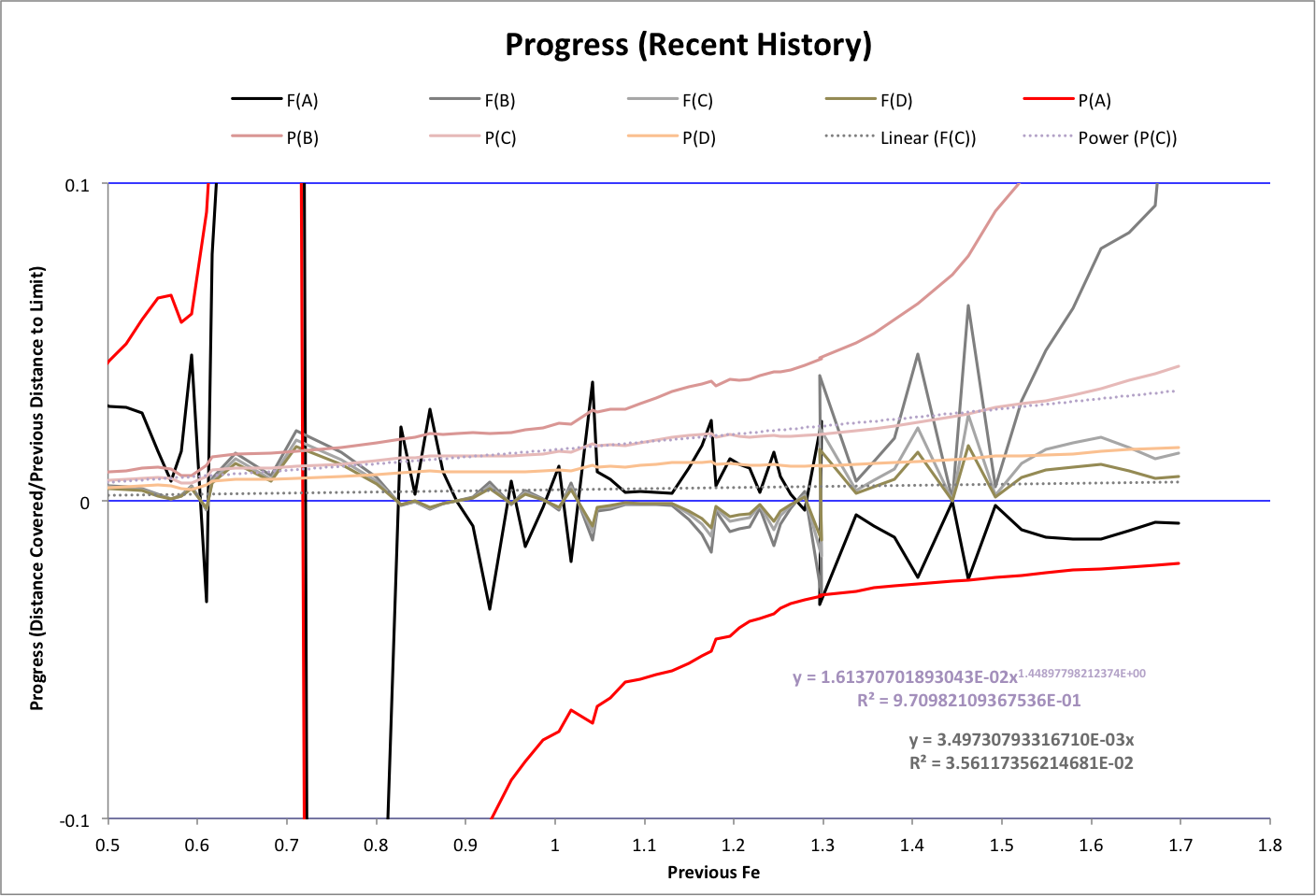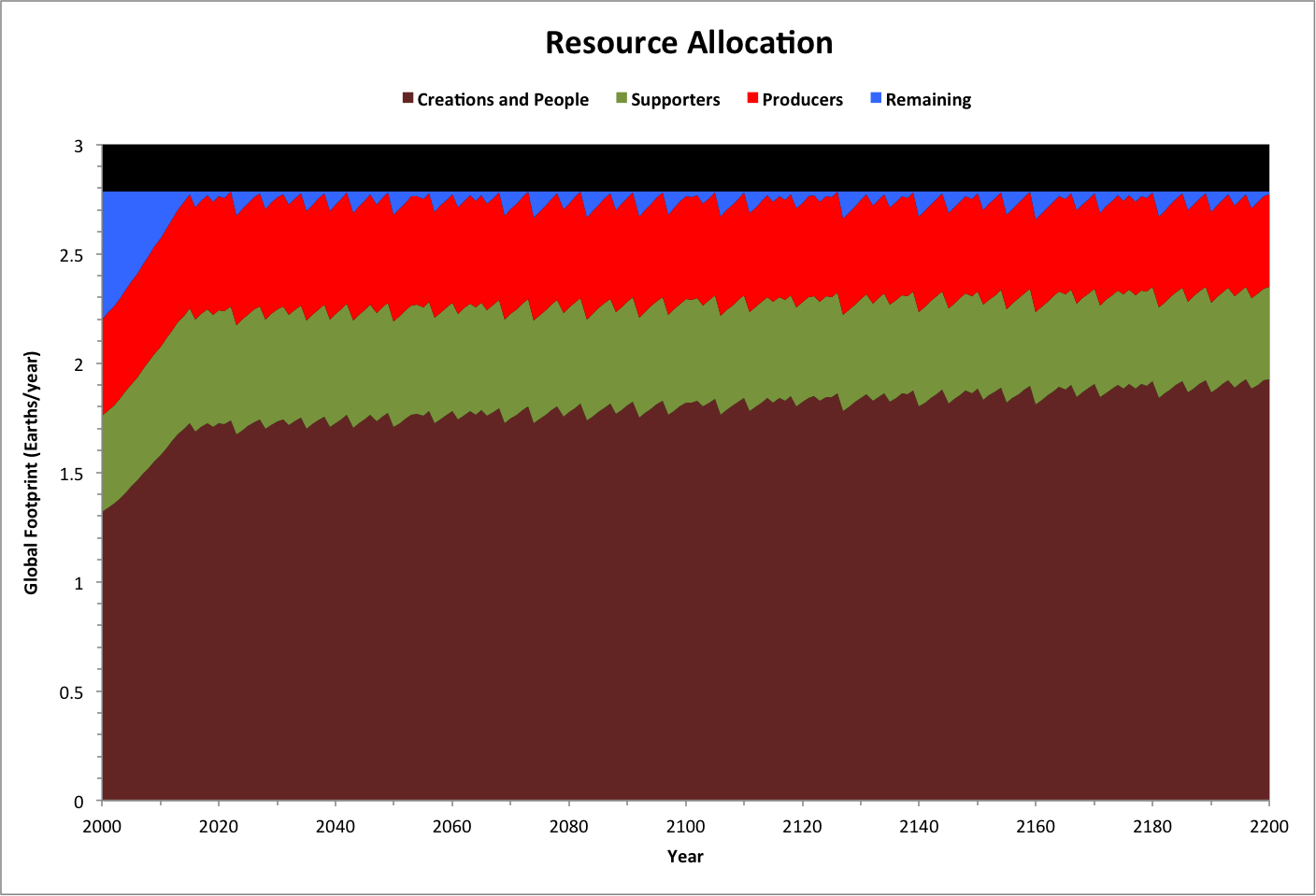Limits

The following limits and conditions may be reached according the Half-Earth Hypothesis (see Priority Interdependencies for variable definitions):

 Range ID Range Conditions A Fe + FeMin < 1 P < 1 / ( F + Fmin) F < ( 1 - P * Fmin ) / P B 1 <= Fe + FeMin < FeTotal - FeMin P < FeTotal  / ( F + 2 * Fmin ) F < FeTotal / P - 2 * Fmin C FeTotal - FeMin < Fe + FeMin < FeTotal P < FeTotal / ( F + Fmin ) F < ( FeTotal - P * Fmin ) / P D Fe = FeTotal P = FeTotal / F F = FeTotal / P

Historically it appears that humanity's population P and footprint F are converging toward the upper limit of range C. This is seen below in a graph of progress toward each upper limit for each of the years 1950-2015. Dotted lines are curve-fits.The following graph shows how ecological resources are distributed over time in terms of global footprint, and using the terminology of the Half-Earth hypothesis.

Note:

• Supporters and producers are each equal to FeMin
• The upper limit of range A  ("limit A") is reached when creations and people (Fe) and supporters (FeMin) together equal 1 Earth/year
• Limit B is reached when there are no remaining resources
• Limit C is reached when there are no producers (producers equal FeMin)
• Limit D is reached when there are no more supporters